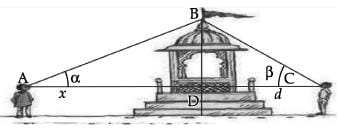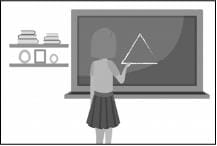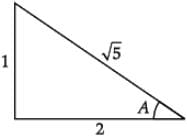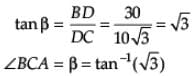Test: Inverse Trigonometric Functions- Case Based Type Questions

# Test: Inverse Trigonometric Functions- Case Based Type Questions

Test Description

## 15 Questions MCQ Test Mathematics (Maths) Class 12 | Test: Inverse Trigonometric Functions- Case Based Type Questions

Test: Inverse Trigonometric Functions- Case Based Type Questions for Commerce 2023 is part of Mathematics (Maths) Class 12 preparation. The Test: Inverse Trigonometric Functions- Case Based Type Questions questions and answers have been prepared according to the Commerce exam syllabus.The Test: Inverse Trigonometric Functions- Case Based Type Questions MCQs are made for Commerce 2023 Exam. Find important definitions, questions, notes, meanings, examples, exercises, MCQs and online tests for Test: Inverse Trigonometric Functions- Case Based Type Questions below.
Solutions of Test: Inverse Trigonometric Functions- Case Based Type Questions questions in English are available as part of our Mathematics (Maths) Class 12 for Commerce & Test: Inverse Trigonometric Functions- Case Based Type Questions solutions in Hindi for Mathematics (Maths) Class 12 course. Download more important topics, notes, lectures and mock test series for Commerce Exam by signing up for free. Attempt Test: Inverse Trigonometric Functions- Case Based Type Questions | 15 questions in 30 minutes | Mock test for Commerce preparation | Free important questions MCQ to study Mathematics (Maths) Class 12 for Commerce Exam | Download free PDF with solutions
 1 Crore+ students have signed up on EduRev. Have you?
Test: Inverse Trigonometric Functions- Case Based Type Questions - Question 1

### Direction: Read the following text and answer the following questions on the basis of the same:Two men on either side of a temple of 30 metres high observe its top at the angles of elevation α and β respectively. (as shown in the figure above). The distance between the two men is 40√3 metres and the distance between the first person A and the temple is 30√3 meters.∠CAB = α =

Detailed Solution for Test: Inverse Trigonometric Functions- Case Based Type Questions - Question 1 In Δ BDA

sin α = BD/AB

= (30√3)2 + 302

= 602

AB = 60 m

Now, sinα = 30/60

Sinα = ½

I.e. ∠CAB = α = sin-1(½)

Test: Inverse Trigonometric Functions- Case Based Type Questions - Question 2

### Direction: Read the following text and answer the following questions on the basis of the same:Two men on either side of a temple of 30 metres high observe its top at the angles of elevation α and β respectively. (as shown in the figure above). The distance between the two men is 40√3 metres and the distance between the first person A and the temple is 30√3 meters.Domain and Range of cos−1 x =

Detailed Solution for Test: Inverse Trigonometric Functions- Case Based Type Questions - Question 2 Since cos x is defined at x = 0, and x = π

Domain of cos−1 x includes -1 and 1

Range of cos−1 x also includes 0 and π

Test: Inverse Trigonometric Functions- Case Based Type Questions - Question 3

### Direction: Read the following text and answer the following questions on the basis of the same:In the school project Sheetal was asked to construct a triangle and name it as ABC. Two angles A and B were given to be equal to tan-1(½) and tan-1(⅓) respectively.The value of sin A is _______.

Detailed Solution for Test: Inverse Trigonometric Functions- Case Based Type Questions - Question 3A = tan-1(½)

⇒ tan A = ½

∴ sin A = 1/√5

Test: Inverse Trigonometric Functions- Case Based Type Questions - Question 4

Direction: Read the following text and answer the following questions on the basis of the same:

In the school project Sheetal was asked to construct a triangle and name it as ABC. Two angles A and B were given to be equal to tan-1(½) and tan-1(⅓) respectively.The third angle, ∠C = _______.

Detailed Solution for Test: Inverse Trigonometric Functions- Case Based Type Questions - Question 4 ∠C = π – (A + B)

= π - (π/4)

= 3π/4

Test: Inverse Trigonometric Functions- Case Based Type Questions - Question 5

Direction: Read the following text and answer the following questions on the basis of the same:

The value of an inverse trigonometric functions which lies in the range of Principal branch is called the principal value of that inverse trigonometric functions.

Principal value of sin–1 (½) is

Detailed Solution for Test: Inverse Trigonometric Functions- Case Based Type Questions - Question 5 sin-1(½) = y

sin y = ½

Principal value branch of sin–1 is (-π/2, π/2)

and sin(π/6) = ½

⇒ Principal value of sin-1(½) is π/6.

Test: Inverse Trigonometric Functions- Case Based Type Questions - Question 6

Direction: Read the following text and answer the following questions on the basis of the same:

The value of an inverse trigonometric functions which lies in the range of Principal branch is called the principal value of that inverse trigonometric functions.

Principal value of 2cos–1 (1) + 5tan–1 (1) is:

Detailed Solution for Test: Inverse Trigonometric Functions- Case Based Type Questions - Question 6 2cos-1(1) + 5tan-1(1)

= 2 x 0 + 5 x π/4

= 5π/4

Test: Inverse Trigonometric Functions- Case Based Type Questions - Question 7

Direction: Read the following text and answer the following questions on the basis of the same:Two men on either side of a temple of 30 metres high observe its top at the angles of elevation α and β respectively. (as shown in the figure above). The distance between the two men is 40√3 metres and the distance between the first person A and the temple is 30√3 meters.

∠CAB = α =

Detailed Solution for Test: Inverse Trigonometric Functions- Case Based Type Questions - Question 7 In Δ BDA

Cosα = 30√3/60

α = cos-1(√3/2)

∴∠CAB = α = cos-1(√3/2)

Test: Inverse Trigonometric Functions- Case Based Type Questions - Question 8

Direction: Read the following text and answer the following questions on the basis of the same:

In the school project Sheetal was asked to construct a triangle and name it as ABC. Two angles A and B were given to be equal to tan-1(½) and tan-1(⅓) respectively.cos(A + B + C) = _______.

Detailed Solution for Test: Inverse Trigonometric Functions- Case Based Type Questions - Question 8 Since ABC is a triangle,

∴ A + B + C = 180°

cos (A + B + C) = cos 180°

= -1

Test: Inverse Trigonometric Functions- Case Based Type Questions - Question 9

Direction: Read the following text and answer the following questions on the basis of the same:

The value of an inverse trigonometric functions which lies in the range of Principal branch is called the principal value of that inverse trigonometric functions.

Principal value of tan–1 (1)

Detailed Solution for Test: Inverse Trigonometric Functions- Case Based Type Questions - Question 9 tan-1(1) =tan-1(tan(π/4))

= π/4

Test: Inverse Trigonometric Functions- Case Based Type Questions - Question 10

Direction: Read the following text and answer the following questions on the basis of the same:Two men on either side of a temple of 30 metres high observe its top at the angles of elevation α and β respectively. (as shown in the figure above). The distance between the two men is 40√3 metres and the distance between the first person A and the temple is 30√3 meters.

∠BCA = β =

Detailed Solution for Test: Inverse Trigonometric Functions- Case Based Type Questions - Question 10 DC = AC - AD

= 40√3 - 30√3

= 10√3 m

In ΔBDCTest: Inverse Trigonometric Functions- Case Based Type Questions - Question 11

Direction: Read the following text and answer the following questions on the basis of the same:

In the school project Sheetal was asked to construct a triangle and name it as ABC. Two angles A and B were given to be equal to tan-1(½) and tan-1(⅓) respectively.If B = cos–1 x, then x = _______.

Detailed Solution for Test: Inverse Trigonometric Functions- Case Based Type Questions - Question 11 Given B = tan-1(⅓)

⇒ tan B = ⅓

∴ cos B = 3/√10

B = cos-1(3/√10)

⇒ x = 3/√10

Test: Inverse Trigonometric Functions- Case Based Type Questions - Question 12

Direction: Read the following text and answer the following questions on the basis of the same:

The value of an inverse trigonometric functions which lies in the range of Principal branch is called the principal value of that inverse trigonometric functions.

Principal value of cot-1(√3) is :

Detailed Solution for Test: Inverse Trigonometric Functions- Case Based Type Questions - Question 12 cot -1(√3) =cot-1 (cot (π/6) = π/6
Test: Inverse Trigonometric Functions- Case Based Type Questions - Question 13

Direction: Read the following text and answer the following questions on the basis of the same:Two men on either side of a temple of 30 metres high observe its top at the angles of elevation α and β respectively. (as shown in the figure above). The distance between the two men is 40√3 metres and the distance between the first person A and the temple is 30√3 meters.

∠ABC =

Detailed Solution for Test: Inverse Trigonometric Functions- Case Based Type Questions - Question 13 Since,

Sin α = ½

i.e., sin α = sin 300 [∵ sin 300 = ½]

∴ α = 300

We have tan β = √3

tan β = tan 600

∴ β = 600

Now In ΔABC

∠ABC + ∠BCA + ∠CAB = 1800

∠ABC + 600 + 300 = 1800

∠ABC = 900

∴ ∠ABC = π/2

Test: Inverse Trigonometric Functions- Case Based Type Questions - Question 14

Direction: Read the following text and answer the following questions on the basis of the same:

In the school project Sheetal was asked to construct a triangle and name it as ABC. Two angles A and B were given to be equal to tan-1(½) and tan-1(⅓) respectively.If A = sin–1x; then the value of x is:

Detailed Solution for Test: Inverse Trigonometric Functions- Case Based Type Questions - Question 14 A = tan-1 (½)

⇒ tan A = ½

∴ Sin A = 1/√5

A = sin-1(1/√5)

⇒ x = 1/√5

Test: Inverse Trigonometric Functions- Case Based Type Questions - Question 15

Direction: Read the following text and answer the following questions on the basis of the same:

The value of an inverse trigonometric functions which lies in the range of Principal branch is called the principal value of that inverse trigonometric functions.

Principal value of sin -1(1) + sin-1(1/√2) is

Detailed Solution for Test: Inverse Trigonometric Functions- Case Based Type Questions - Question 15 sin-1(1) + sin-1(1/√2) = π//2 + π/4

= 3π/4

## Mathematics (Maths) Class 12

209 videos|218 docs|139 tests
 Use Code STAYHOME200 and get INR 200 additional OFF Use Coupon Code
Information about Test: Inverse Trigonometric Functions- Case Based Type Questions Page
In this test you can find the Exam questions for Test: Inverse Trigonometric Functions- Case Based Type Questions solved & explained in the simplest way possible. Besides giving Questions and answers for Test: Inverse Trigonometric Functions- Case Based Type Questions, EduRev gives you an ample number of Online tests for practice

## Mathematics (Maths) Class 12

209 videos|218 docs|139 tests